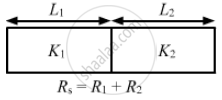Advertisement Remove all ads

# A Composite Slab is Prepared by Pasting Two Plates of Thickness L1 and L2 and Thermal Conductivites K1 and K2. the Slabs Have Equal Cross-sectional Area. Find the Equivalent Conductivity of the - Physics

Sum

A composite slab is prepared by pasting two plates of thickness L1 and L2 and thermal conductivites K1 and K2. The slabs have equal cross-sectional area. Find the equivalent conductivity of the composite slab.

Advertisement Remove all ads

#### SolutionIt is equivalent to the series combination of 2 resistors.

∴ RS = R1 +R2

Resistance of a conducting slab,  R = l/(KA)

(L_1 + L_2)/(K_SA)=(L_1)/(K_1A)+ (L_2)/(K_2A)

(L_1 +L_2)/(K_s) = L_1/K_1 + L_2/K_2

(L_1 + L_2 )/K_s = (L_1K_2 + L_2K_1)/(K_1 xx K_2)

K_s = ((L_1 + L_2) (K_1 K_2))/(L_1k_2 + L_2K_1

Concept: Thermal Expansion of Solids
Is there an error in this question or solution?
Advertisement Remove all ads

#### APPEARS IN

HC Verma Class 11, Class 12 Concepts of Physics Vol. 2
Chapter 6 Heat Transfer
Q 22 | Page 99
Advertisement Remove all ads
Advertisement Remove all ads
Share
Notifications

View all notifications

Forgot password?